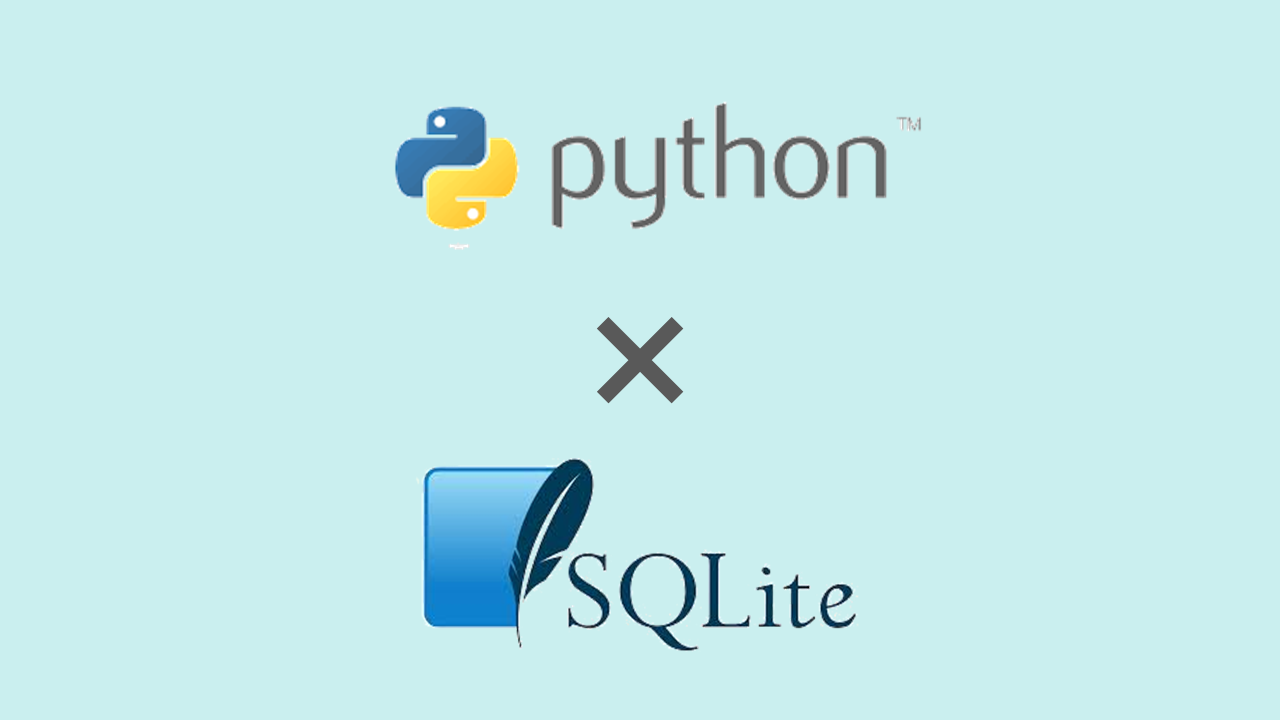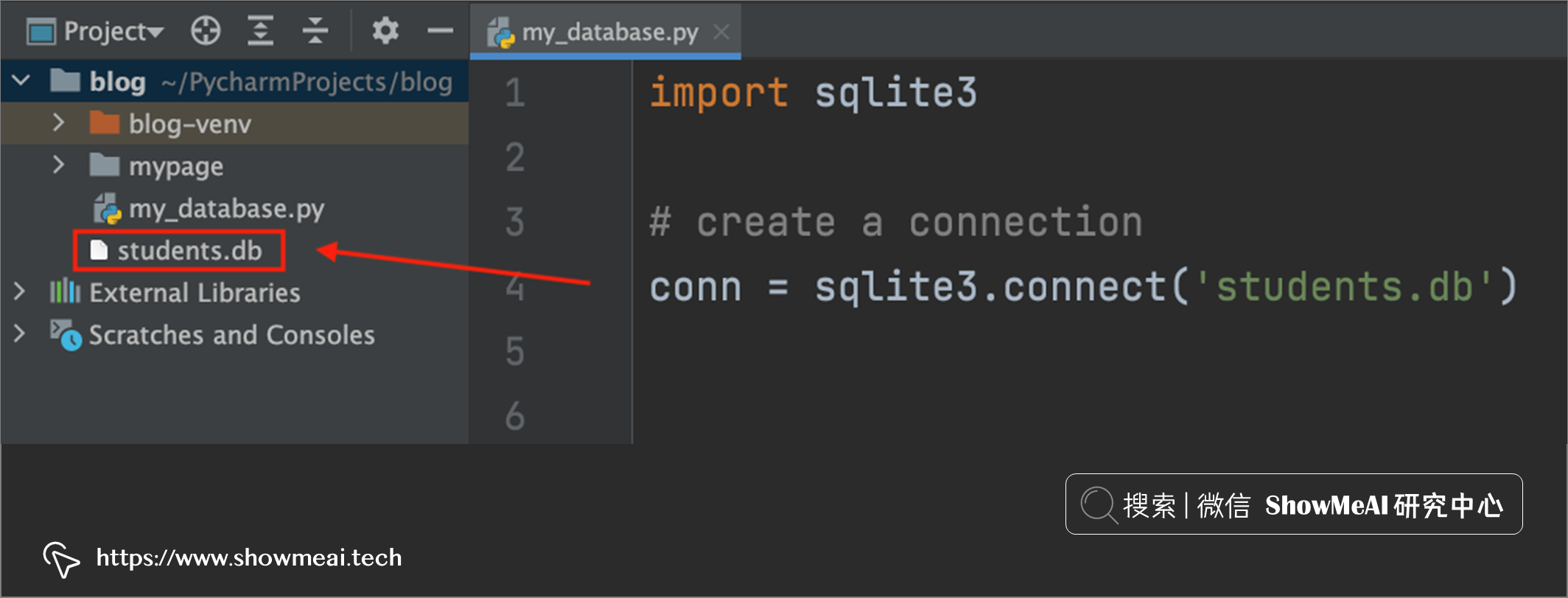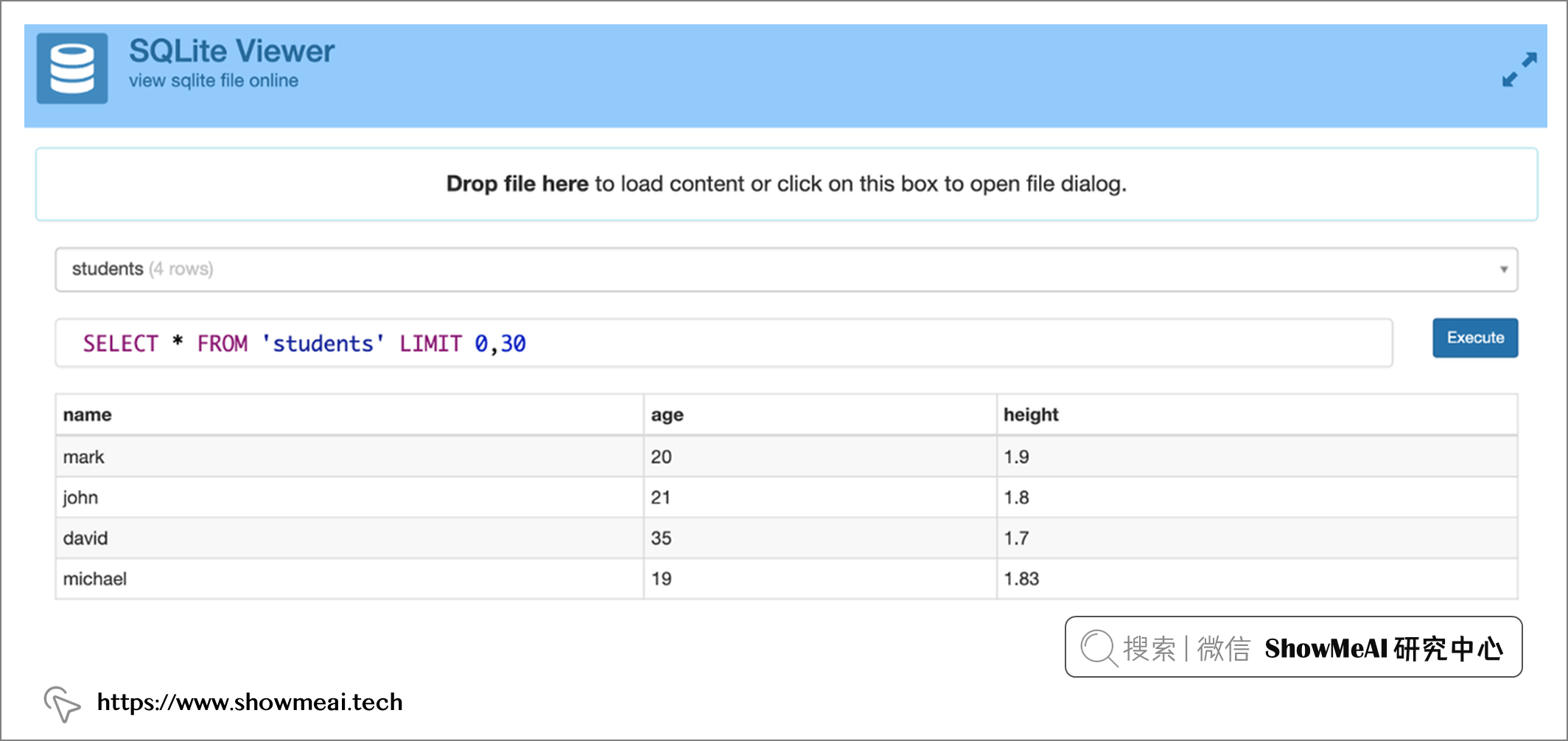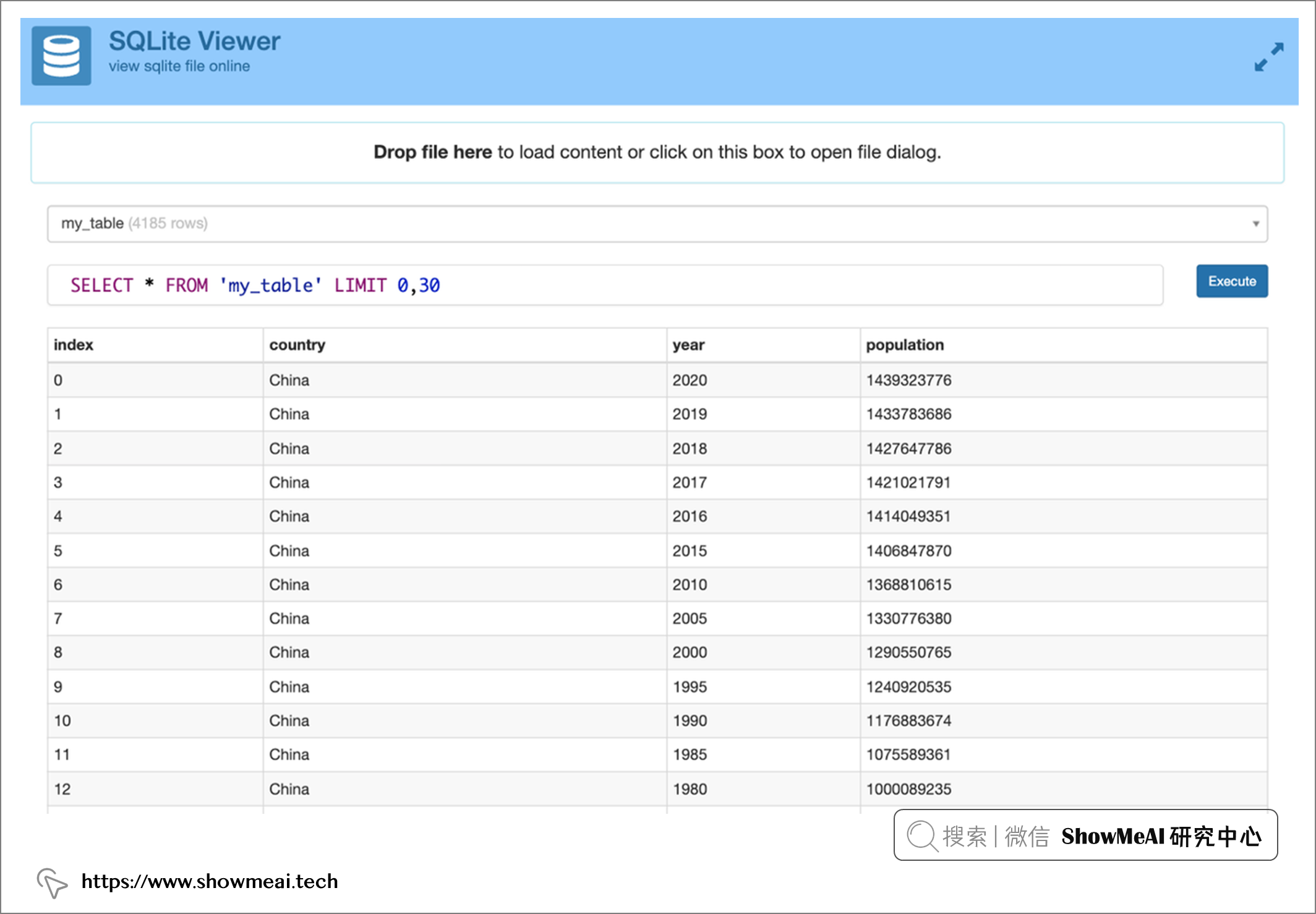# Python中內置數據庫！SQLite使用指南！

## highlight: a11y-darkPython 是一個廣泛使用的編程語言，在各個領域都能發揮很大的作用，而且安裝 Python 環境的同時，我們也安裝了很多其他出色的工具，其中當然少不了數據庫。

Python 內置了 SQLite3，在 Python 中使用 SQLite，不需要安裝任何東西，可以直接使用。我們只需要導入內置 Python 庫`sqlite3`就可以開始使用這個數據庫啦！```python

import sqlite3

# 建立連接

conn = sqlite3.connect('students.db') ```# 💡 創建表

```python c = conn.cursor()```

```python c.execute("""CREATE TABLE students ( name TEXT, age INTEGER, height REAL )""")```

• `Null`：缺失值
• `INTEGER`：沒有小數點的數字（例如，1、2、3、4）
• `REAL`：帶小數點的數字（例如，6.2、7.6、11.2）
• `TEXT`：任何字符數據
• `Blob`：二進制數據的集合，作為值存儲在數據庫中。它允許我們在數據庫中存儲文檔、圖像和其他多媒體文件。

```python

import sqlite3

# 創建連接

conn = sqlite3.connect('students.db')

# 遊標

c = conn.cursor()

# 建表語句

c.execute("""CREATE TABLE students ( name TEXT, age INTEGER, height REAL )""")

conn.commit()

conn.close() ```

# 💡 插入數據

```python c.execute("INSERT INTO students VALUES ('mark', 20, 1.9)")```

```python all_students = [ ('john', 21, 1.8), ('david', 35, 1.7), ('michael', 19, 1.83), ] c.executemany("INSERT INTO students VALUES (?, ?, ?)", all_students)```

# 💡 查詢數據

```python c.execute("SELECT * FROM students") print(c.fetchall())```

```python [(‘mark’, 20, 1.9), (‘john’, 21, 1.8), (‘david’, 35, 1.7), (‘michael’, 19, 1.83)]``````python

import sqlite3

# 創建連接

conn = sqlite3.connect('students.db')

# 遊標

c = conn.cursor()

# 建表語句

c.execute("""CREATE TABLE students ( name TEXT, age INTEGER, height REAL )""")

# 插入單條數據

c.execute("INSERT INTO students VALUES ('mark', 20, 1.9)")

# 插入多條數據

all_students = [ ('john', 21, 1.8), ('david', 35, 1.7), ('michael', 19, 1.83), ] c.executemany("INSERT INTO students VALUES (?, ?, ?)", all_students)

# 查詢數據

c.execute("SELECT * FROM students") print(c.fetchall())

conn.commit()

conn.close() ```

# 💡 SQLite 配合 Pandas 應用

SQLite 可以與 Pandas 中的Dataframe搭配使用。

🏆 實戰數據集下載（百度網盤）：公眾號『ShowMeAI研究中心』回覆『實戰』，或者點擊 這裏 獲取本文 Python內置數據庫SQLite使用指南SQLite示例數據集

ShowMeAI官方GitHubhttps://github.com/ShowMeAI-Hub

```python import pandas as pd df = pd.read_csv("population_total.csv")```

Dataframe 內容如下所示：

```python

df country year population0 China 2020.0 1.439324e+09 1 China 2019.0 1.433784e+09 2 China 2018.0 1.427648e+09 3 China 2017.0 1.421022e+09 4 China 2016.0 1.414049e+09 ... ... ... ... 4180 United States 1965.0 1.997337e+08 4181 United States 1960.0 1.867206e+08 4182 United States 1955.0 1.716853e+08 4183 India 1960.0 4.505477e+08 4184 India 1955.0 4.098806e+08 ```

```python from sqlalchemy import create_engine engine = create_engine('sqlite://', echo=False)```

```python df.to_sql("population", con=engine)```

```python engine.execute("SELECT * FROM population").fetchall()```

```python from sqlalchemy import create_engine engine = create_engine("sqlite:///mydb.db") df.to_sql("population", engine)```# 參考資料